# An Introduction to Integers

Go back to  'Integers'

## Introduction to Integers

Till now all mathematical operations have been performed on whole or natural numbers. Working with numbers beginning from zero and progressing towards the right of the number line. What exists on the left of the number line?## What are integers?

Up to this point, children could answer questions like which number is smaller than 2 (ans: 0 or 1). However, what if they were asked what is smaller than zero? Therefore, the need arises to introduce a completely new group of numbers called negative numbers.

Integers are numbers that are whole numbers and negative numbers.

All integers are represented by the alphabet Z and are written as;

Z = {…, –4, –3, –2, –1, 0, 0, 1, 2, 3, 4, …}Numbers that are on the left of zero are denoted by a negative () sign, thus making them negative integers.
Numbers that are on the right of zero are positive integers.
Numbers to the left represent a decrease and numbers to the right represent an increase.
Integer numbers cannot have decimals and cannot be fractions

Numbers that are integers

$$-20, 45, -998, 26, 0$$

Numbers that are not integers

$$-1.23,$$ $${6 \over 8},$$ $$89.75$$

What about something like $${30 \over 6}$$? Well, at first it may look at bit odd, but if you remember the principles of simplification of fractions, it comes out to be 5, which is an integer!

All mathematical operations such as addition, subtraction, division and multiplication can be performed on integers. All mathematical operations on integers can be solved by following a few simple guidelines.

If both numbers have the same sign, add both numbers and include the same sign as both.

Example:

1. 5 + 6 = 11
2. –6 + (–9) = –15

If both numbers have different signs, add or subtract the number and include the sign of the largest absolute integer.

Example:

1. 9 + (–5)
a. 9 –
5
b. 4
2. –9 + 5
a. –4
b. –9 is larger than 5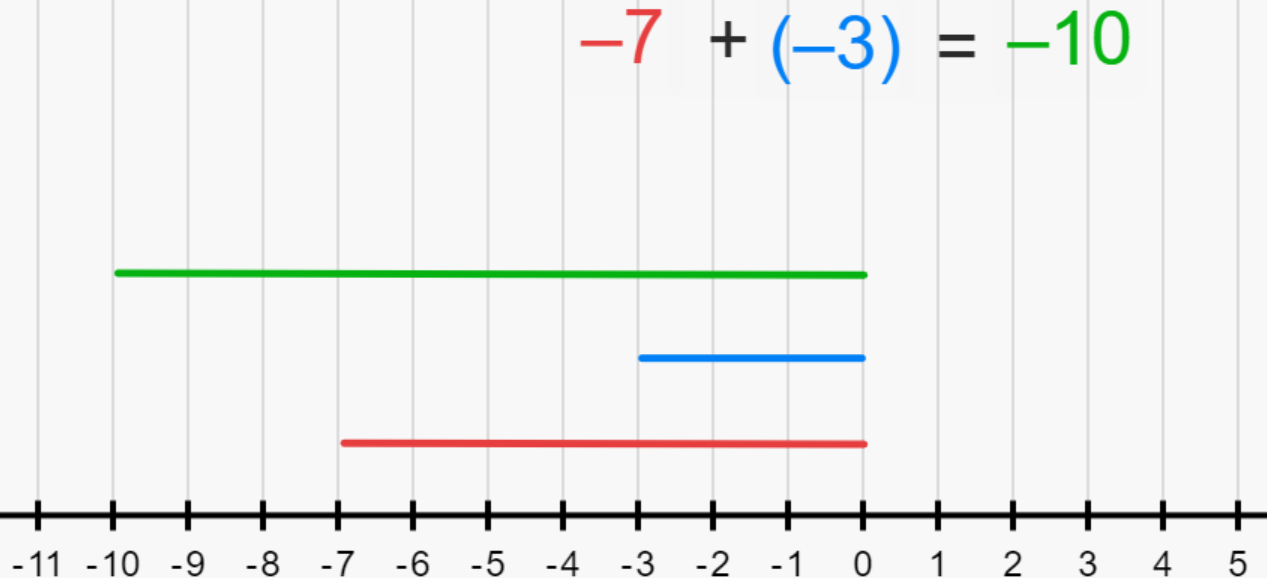### Subtraction of integers

If both numbers have positive signs, the difference will be positive.

Example:

10 – 5 = 2

If both numbers have a negative sign, subtract the two numbers and include the sign of the largest absolute value.

Example:

1. –10 – (–25)
a. –10 + 25 according to the rules of multiplication 2 negatives make a positive.
b. 15
25 is larger than 10
2. –25 – (–10)
a. –25 + 10
2 negatives make a positive
b. –15
25 is larger than 15

If both numbers have different signs, subtract the two and denote the difference with the sign of the largest absolute value.

Example:

1. 18 – 5 = 13
18 is larger than 3 and has a positive value
2. –3 – 18 = –21
2 negative signs call for addition
3. 3 – 18 = –15
18 is larger than 3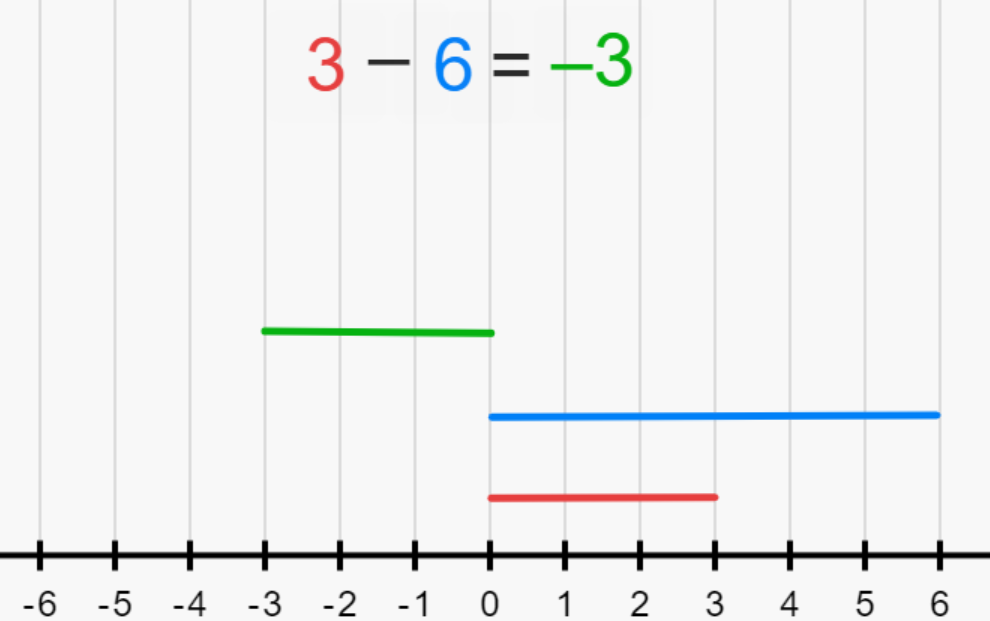### Multiplication of integers

If both integers have the same sign, then the product is positive.

Example:

1. 2 x 5 = 10
2. –2 x –5 = 10

If both integers have different signs, the product is always negative.

Example:

1. 2 x –5 = –10
2. –2 x 9 = –18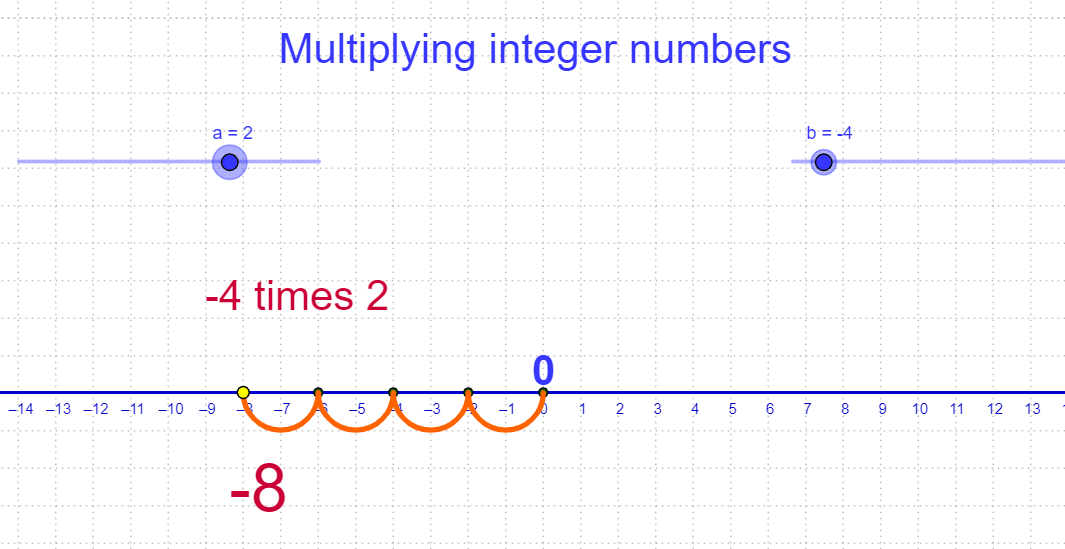### Division of integers

If both integers have the same sign, then the quotient is positive.

Example:

1. 16 ÷ 8 = 2
2. –16 ÷ –8 = 2

If both integers have different signs, the quotient is always negative.

Example:

1. –16 ÷ 8 = –2
2. 10 ÷ –2 = –5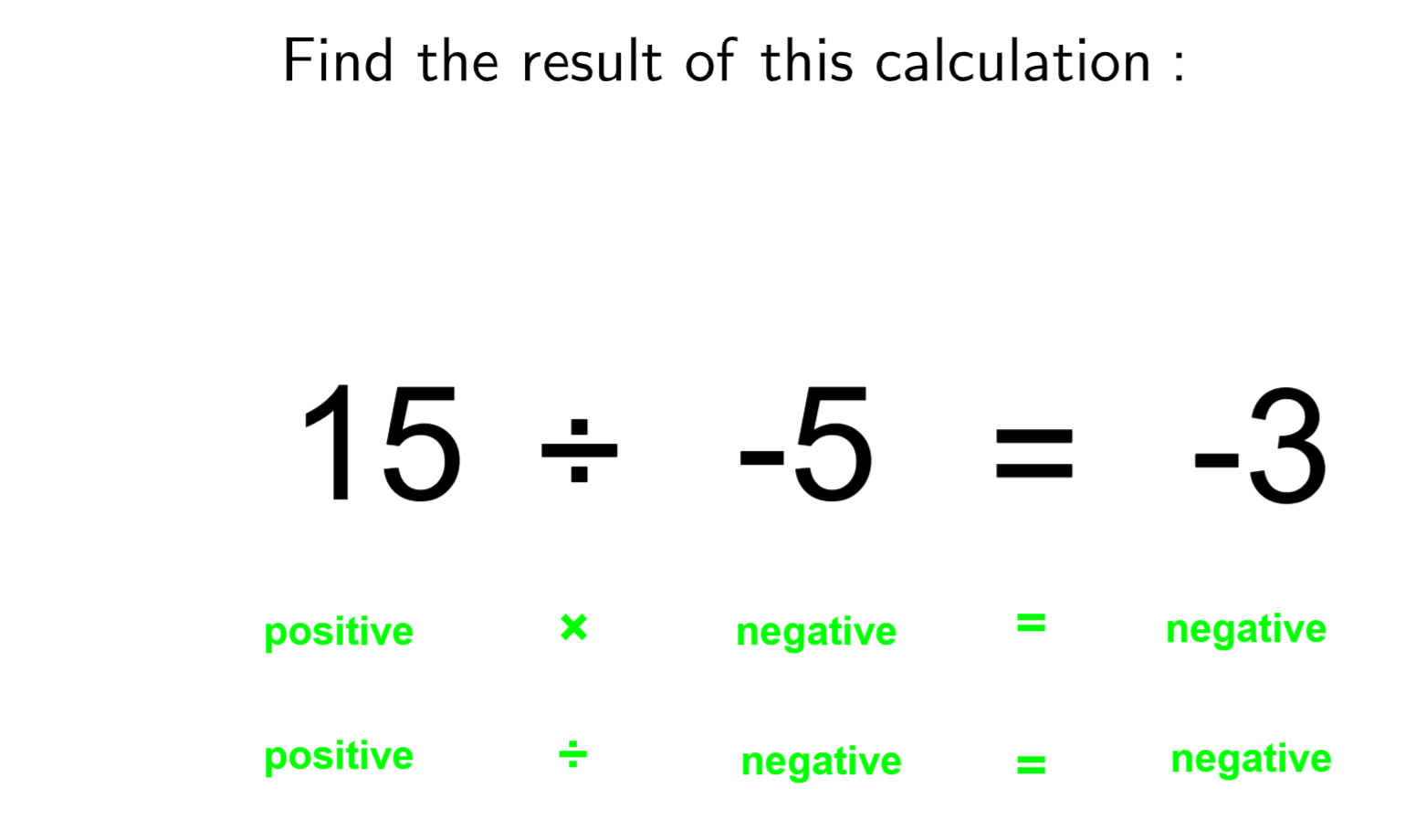## Why is it important?

### The concept of a number line

All mathematical operations on integers are easier to understand once there is a strong understanding of the number line.

Example:

6 – 3 = 3

1. Step 1: we go three places to the right of 0, landing on to 6.
2. Step 2: since the operation calls for a subtraction of 3, from the 6 we move 3 steps further to the left.
3. Step 3: now we are on 3. Hence proving the addition and the utilization of the number line.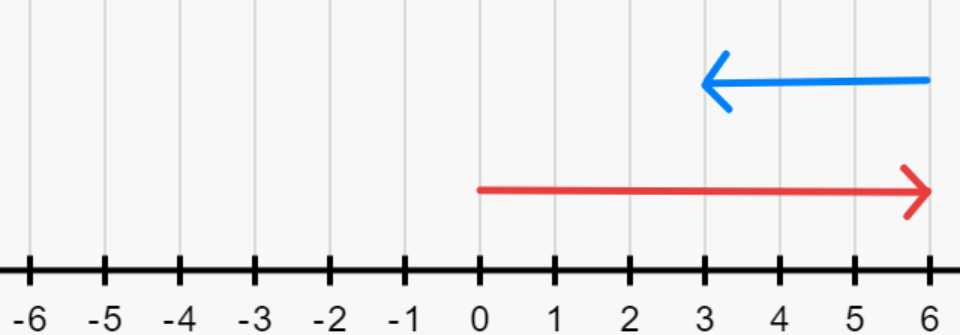### Zeros and fractions

When it comes to integers, the number 0 is neither positive or negative. It is a counting number which symbolises that there are no objects.

Children often make errors in judgement when it comes to fractions. According to the rules, an integer cannot be a fraction. But the fraction $${25 \over 5}$$ is, in fact, an integer. 5 x 5 = 25.  25 ÷ 5 = 5. Reducing it to a whole number, making it an integer. With the rules of the division, children will discover that when fractions are further reduced to a number without any remainders, they are integers.

### Integers and the real world

Integers and their properties are not explicit only to the classrooms. They are everywhere around us, in our everyday activities. Anything that is increasing is positive, and anything with a decreasing factor is negative.

• In the bank account, every time money is deposited, is positive. Nut, when money is withdrawn the balance, decreases making with withdrawal a negative.
• With the rise in temperature during summers, it is denoted with a positive sign. But in the cold of the winters, temperature decreases and is denoted by a negative sign.
• In business, with profit, the numbers are in positive, and losses are negative. With exercise, calories gained are positive and calories lost are negative

Having a core understanding of the number line and a strong handle on the rules of operation on integers simplifies mathematics in general.

However, we at Cuemath, are driven to put the Why before the what and ensure that in our classes, the concepts come first.